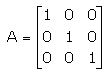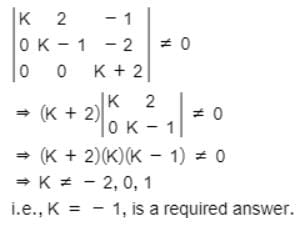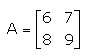JEE  >  Test: Solving Simultaneous Equations

# Test: Solving Simultaneous Equations

Test Description

## 10 Questions MCQ Test Mathematics (Maths) Class 12 | Test: Solving Simultaneous Equations

Test: Solving Simultaneous Equations for JEE 2023 is part of Mathematics (Maths) Class 12 preparation. The Test: Solving Simultaneous Equations questions and answers have been prepared according to the JEE exam syllabus.The Test: Solving Simultaneous Equations MCQs are made for JEE 2023 Exam. Find important definitions, questions, notes, meanings, examples, exercises, MCQs and online tests for Test: Solving Simultaneous Equations below.
Solutions of Test: Solving Simultaneous Equations questions in English are available as part of our Mathematics (Maths) Class 12 for JEE & Test: Solving Simultaneous Equations solutions in Hindi for Mathematics (Maths) Class 12 course. Download more important topics, notes, lectures and mock test series for JEE Exam by signing up for free. Attempt Test: Solving Simultaneous Equations | 10 questions in 10 minutes | Mock test for JEE preparation | Free important questions MCQ to study Mathematics (Maths) Class 12 for JEE Exam | Download free PDF with solutions
 1 Crore+ students have signed up on EduRev. Have you?
Test: Solving Simultaneous Equations - Question 1

### The solution of the following system of equation is 2x + 3y = 5 5x – 2y = 3​

Detailed Solution for Test: Solving Simultaneous Equations - Question 1

2x + 3y = 5
Multiply equation with ‘5’, we get
10x + 15y = 25……………………(1)
5x – 2y = 3
Multiply equation with ‘2’, we get
10x - 4y = 6………………………(2)
Subtracting (1) from (2), we get
19y = 19
y = 1
Put the value of y in eq(1)
10x + 15(1) = 25
10x = 10
x = 1

Test: Solving Simultaneous Equations - Question 2

### One third of sum of two angles is 60° and one fourth of their difference is 28°. The angles are

Detailed Solution for Test: Solving Simultaneous Equations - Question 2

Solution:-
Let the two angles be 'x' and 'y'.
So, according to the question,
One third of the sum of two angles :- 1/3(x+y) = 60
x/3 + y/3 = 60    ......(1)
Quarter of their difference :- 1/4(x-y) = 28
x/4 - y/4 = 28...........(2)
Multiplying the equation (1) by 3 and equation (2) by 4, we get
x + y =180  .......(3)   x - y = 112  ......(4)
Subtracting (4) from (3),
x + y = 180
x - y = 112
-   +     -
_________
2y = 68
_________
2y = 68
y = 34
Putting the value of y = 34 in the equation (3)
x + y = 180
x + 34 = 180
x = 180 - 34
x = 146
The two angles are 146° and 34°.

Test: Solving Simultaneous Equations - Question 3

### For a square matrix A in a matrix equation AX = B, if │A│≠ 0, then​

Test: Solving Simultaneous Equations - Question 4

The following system of equations has
x + 3y + 3z = 2
x + 4y + 3z = 1
x + 3y + 4z = 2​

Detailed Solution for Test: Solving Simultaneous Equations - Question 4

Let A = {(1,3,3) (1,4,3) (1,3,4)}
|A| = 1(16-9) -3(4-3) +3(3-4)
|A| = 1(7) -3(1) +3(-1)
= 7 - 3 - 3
= 1
Therefore, A is not equal to zero, it has unique solution.

Test: Solving Simultaneous Equations - Question 5

Inverse of a matrix A is given by

Detailed Solution for Test: Solving Simultaneous Equations - Question 5

Inverse of matrix (A-1) = (adj A)/|A|

Test: Solving Simultaneous Equations - Question 6

If, then A-1 =

Test: Solving Simultaneous Equations - Question 7

The system of equations kx + 2y – z = 1,
(k – 1)y – 2z = 2
(k + 2)z = 3 has a unique solution, if k is

Detailed Solution for Test: Solving Simultaneous Equations - Question 7

This system of equations has a unique solution, ifTest: Solving Simultaneous Equations - Question 8

System of equations AX = B is inconsistent if​

Detailed Solution for Test: Solving Simultaneous Equations - Question 8

If (adj A) B ≠ 0 (zero matrix), then the solution does not exist. The system of equations is inconsistent. Else, if (adj A) B = 0 then the system will either have infinitely many solutions (consistent system) or no solution (inconsistent system).

Test: Solving Simultaneous Equations - Question 9

Inverse of, is

Detailed Solution for Test: Solving Simultaneous Equations - Question 9

A = {(6,7) (8,9)}
|A| = (6 * 9) - (8 * 7)
= 54 - 56
|A| = -2
A-1 = -½{(9,-7) (-8,6)}
A-1 = {(-9/2, 7/2) (4,-3)}

Test: Solving Simultaneous Equations - Question 10

A system of linear equations AX = B is said to be inconsistent, if the system of equations has​

Detailed Solution for Test: Solving Simultaneous Equations - Question 10

A linear system is said to be consistent if it has at least one solution; and is said to be inconsistent if it has no solution. have no solution, a unique solution, and infinitely many solutions, respectively.

## Mathematics (Maths) Class 12

209 videos|218 docs|139 tests
Information about Test: Solving Simultaneous Equations Page
In this test you can find the Exam questions for Test: Solving Simultaneous Equations solved & explained in the simplest way possible. Besides giving Questions and answers for Test: Solving Simultaneous Equations , EduRev gives you an ample number of Online tests for practice

## Mathematics (Maths) Class 12

209 videos|218 docs|139 tests(Scan QR code)